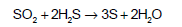Courses

# Mole Concept (Chapter Test - Medical)

## 20 Questions MCQ Test | Mole Concept (Chapter Test - Medical)

Description
This mock test of Mole Concept (Chapter Test - Medical) for Class 12 helps you for every Class 12 entrance exam. This contains 20 Multiple Choice Questions for Class 12 Mole Concept (Chapter Test - Medical) (mcq) to study with solutions a complete question bank. The solved questions answers in this Mole Concept (Chapter Test - Medical) quiz give you a good mix of easy questions and tough questions. Class 12 students definitely take this Mole Concept (Chapter Test - Medical) exercise for a better result in the exam. You can find other Mole Concept (Chapter Test - Medical) extra questions, long questions & short questions for Class 12 on EduRev as well by searching above.
QUESTION: 1

Solution:
QUESTION: 2

Solution:
QUESTION: 3

### A compound contains 8% sulphur. The minimum molecular weight of the compound is

Solution:
QUESTION: 4

Which of the following has the smallest number of molecules?

Solution:
QUESTION: 5

Number of electrons present in 3.6 mg of NH4+ are

Solution:
QUESTION: 6

A sample of CaCO3 has Ca = 40%, C = 12% & O = 48%. If the law of constant proportions is true , then the mass of Ca in 5g of CaCO3 from another source will be

Solution:
QUESTION: 7

One amu is

Solution:
QUESTION: 8

The number of molecules present in a drop of water, if its volume is 0.045 mL, are

Solution:
QUESTION: 9

A hydrate of Na2SO3 losses 22.2% of H2O by mass on strong heating. The hydrate is

Solution:
QUESTION: 10

How many moles of magnesium phosphate, Mg3(PO4)2 will contain 0.25 mole of oxygen atoms?

Solution:
QUESTION: 11

If NA is Avogadro’s number, then number of valence electrons in 4.2 g of nitride ions (N3–)

Solution:
QUESTION: 12

The maximum number of molecules are present in

Solution:
QUESTION: 13

2 moles of H2S and 11.2 L of SO2 at NTP, react to form X mole of sulphur. X isSolution:
QUESTION: 14

The weight of 1 mole of a gas of density 0.1784 g/L at NTP is

Solution:
QUESTION: 15

The law of multiple proportions is illustrated by the two compounds

Solution:
QUESTION: 16

For the formation of 3.65 g of hydrogen chloride gas, what volumes of hydrogen gas and chlorine gas are required at NTP conditions ?

Solution:
QUESTION: 17

The total number of protons, electrons and neutrons in 12 g of C12 is

Solution:
QUESTION: 18

What volume of hydrogen will be liberated at NTP by the reaction of Zn on 50 ml of dilute H2SO4 of density 1.3/ml and having purity 40% ?

Solution:
QUESTION: 19

The number of H+ ions present in 100 mL of 0.001 M H2SO4 solution is

Solution:
QUESTION: 20

The amount of wet NaOH containing 20% water by mass required to neutralise 6 litre of 0.5 M H2SO4 solution is

Solution: